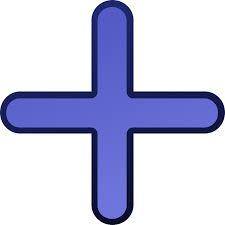# Numbers 3974

The sum of two numbers is 10,000, and one is four times larger than the other. What are two numbers?

a =  8000
b =  2000

### Step-by-step explanation:

a+b=10000
a = 4b

a+b=10000
a = 4·b

a+b = 10000
a-4b = 0

Row 2 - Row 1 → Row 2
a+b = 10000
-5b = -10000

b = -10000/-5 = 2000
a = 10000-b = 10000-2000 = 8000

a = 8000
b = 2000

Our linear equations calculator calculates it.Did you find an error or inaccuracy? Feel free to write us. Thank you!

Tips for related online calculators
Do you have a linear equation or system of equations and looking for its solution? Or do you have a quadratic equation?